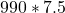## WILL AWARD BRAINLIEST. How many gallons of water fill a tank that is 16.5 ft long, 7.5 ft wide, and 8 ft high? Assume 1 ft3 is 7.5 gallons o

Question

WILL AWARD BRAINLIEST. How many gallons of water fill a tank that is 16.5 ft long, 7.5 ft wide, and 8 ft high? Assume 1 ft3 is 7.5 gallons of water.

in progress 0
6 months 2021-07-24T18:48:24+00:00 1 Answers 2 views 0

## Answers ( )

7,425 gallons of water

Step-by-step explanation:

Given:

A tank of,

Length = 16.5 ft

Width = 7.5 ft

Height = 8 ft

1 ft³ = 7.5 gallons of water

Required:

Gallons of water that will fill the tank

SOLUTION:

Find the volume of the tank:

Volume of the tank = length*width*height

Volume = 16.5*7.5*8 = 990 ft³

Convert 990 ft³ to gallons of water:

1 ft³ = 7.5 gallons

990 ft³ == 7,425 gallons of water

7,425 gallons of water will fill the tank of the given measurement.